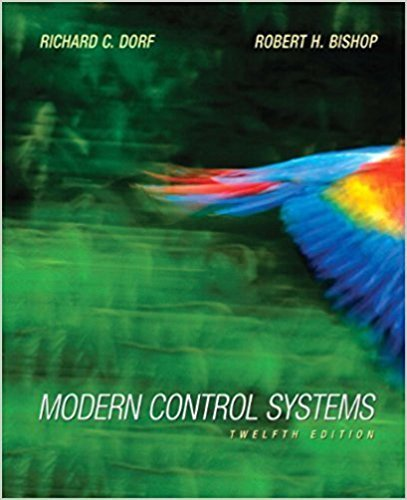Modern Control Systems - 12 Edition - Chapter 4 - Problem E4.3
Register Now

Join StudySoup

Get Full Access to Modern Control Systems - 12 Edition - Chapter 4 - Problem E4.39780136024583

# A robotic arm and camera could be used to pick fruit, as shown in Figure E4.3(a). The

Modern Control Systems | 12th Edition

Problem E4.3

A robotic arm and camera could be used to pick fruit, as shown in Figure E4.3(a). The camera is used to close the feedback loop to a microcomputer, which controls the arm [8,9]. The transfer function for the process is G(s) = K (s + 5)2 (a) Calculate the expected steady-state error of the gripper for a step command A as a function of K. (b) Name a possible disturbance signal for this system.

Accepted Solution
Step-by-Step Solution:
Step 1 of 3

Notes SES100 Phillip Christensen 10/14/2016 A. Matrices a. Computers work with arrays, or matrices of numbers i. Row Matrix ii. Ex. - (1,2,3,4 etc) iii. Ex. - iv. Matrix - 5 Rows & 4 Columns v. To refer to an element in matrix 1. Matlab: It is by row and column a. B = Row 3, column 2 b. a(3,2) c. B = a(3,2) - c​ ommand for MatLab B. Strings a. a = ‘hello’ b. Enclose strings in single quotes c. display(a) d. What would be the value of b after:

###### Chapter 4, Problem E4.3 is Solved

Step 2 of 3

Step 3 of 3

Unlock Textbook Solution